### Product of univariate polynomials

Shoup  showed that the product of univariate polynomials with large degrees and large coefficients can be computed efficiently by FFT over small finite fields and Chinese remainder theorem. It can be easily parallelized:


Input :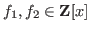such thatOutput :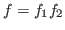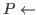where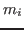is an odd prime,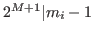andis sufficiently large.

Separate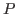into disjoint subsets.

forto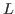Computesuch that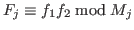andin parallel.
(The product is computed by FFT.)

return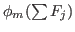(For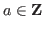,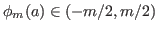and)


Figure 3 shows the speedup factor under the above distributed computation on Risa/Asir. For each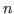, two polynomials of degreewith 3000bit coefficients are generated and the product is computed. The machine is FUJITSU AP3000, a cluster of Sun workstations connected with a high speed network and MPI over the network is used to implement OpenXM.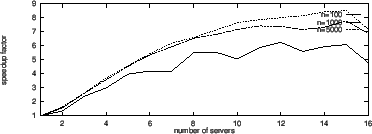If the number of servers isand the inputs are fixed, then the cost to computein parallel is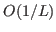, whereas the cost to send and receive polynomials is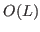if ox_push_cmo() and ox_pop_cmo() are repeatedly applied on the client. Therefore the speedup is limited and the upper bound of the speedup factor depends on the ratio of the computational cost and the communication cost for each unit operation. Figure 3 shows that the speedup is satisfactory if the degree is large andis not large, say, up to 10 under the above environment. If OpenXM provides collective operations for broadcast and reduction such as MPI_Bcast and MPI_Reduce respectively, the cost of sending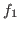,and gatheringmay be reduced to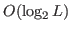and we can expect better results in such a case. In order to implement such operations we need new specifications for inter-sever communication and the session management, which will be proposed as OpenXM-RFC 102. We note that preliminary experiments show the collective operations work well on OpenXM.

Nobuki Takayama 2017-03-30